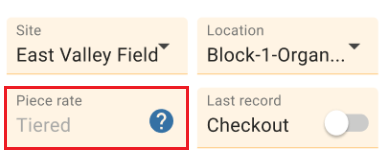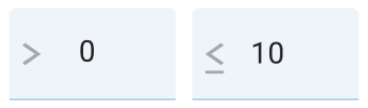# How Do I View & Read the Tiered Piece Rate details

In the Production module, when a tiered piece rate is applied, an information icon appears in the piece rate sectionClick on this icon to display the breakdown of the tiered piece rate tied to the selected pack style

#### How to read the Tiered Piece Rate modal

The “>” symbol means greater than. The “≤” means less than or equal to. Within the tiered piece-rate builder, the symbols are used to convey the floor and cap of the tier.

Tier Example:“>0” means anything above zero. For example, that could be “0.00001”. This is the floor or the bottom of the tier.

“≤10” means 10 or anything less is the cap or the top of the tier. With this cap in place, 10.01 would fall into the second tier.

#### Tiered Piece Rate Types

There are 2 types of tiered piece rates

• Per Unit (Total) - Unit Total Following Tiers
• Per Hour (Avg) - Average Units/Hour Following Tiers

When you click on the help icon in the device, you will see the tiered piece-rate type on top of the tiers

##### Unit Total following Tiers

In this structure type, the system applies the rate that is applicable to each tier band. The total is the combined total across all tiers

 Tier # From unit/hr To unit/hr Each unit paid at 1 >0 ≤10 \$1 2 >10 ≤20 \$2 3 >20 \$3

Example Scenario:

John worked four hours:

• Hour 1 = 5 cases
• Hour 2 = 7 cases
• Hour 3 = 4 cases
• Hour 4 = 6 cases

Completes a total of 22 cases

With a total of 22 cases, based on the tiers he gets:

• First 10 at \$1/ea = \$10 (1-10)
• Second 10 at \$2/ea = \$20 (11-20)
• 21st, and 22nd cases at tier three so 2 at \$3/ea = \$6 (20-22)
• Total of \$36
• (10*1)+(10*2)+(2*3)
##### Average Units/Hour Following Tiers

In this structure, the system utilizes the average number of units produced during the day to determine which piece-rate to apply to all units produced. Within the tiered piece-rate builder, this structure is titled “Avg Units/Hr Following Unit Total”.

 Tier # From unit/hr To unit/hr Each unit paid at 1 >0 ≤20 \$.50 2 >20 ≤40 \$.75 3 >40 \$1

Example Scenario:

Jose worked four hours:

• Hour 1 = 16 vines pruned
• Hour 2 = 22 vines pruned
• Hour 3 = 30 vines pruned
• Hour 4 = 20 vines pruned

Avg units per hour: (16+22+30+20) / 4 = 22

Total # of units generated: 88

Jose’s pay would be calculated as followed:

• Avg vines pruned across the four hours he worked was 22 and that falls within tier #2
• This means that all 88 vines are paid at \$.75 per vine

Jose’s total pay for the four hours he worked would be \$66 (.75*88)

Calificar artículo Calificacion de éxito del artículo Falla en calificación del artículo

Todavia necesita ayuda? Contactanos Contactanos